Courses

# NCERT Exemplar - Arithmetic Progressions Class 10 Notes | EduRev

## Mathematics (Maths) Class 10

Created by: Indu Gupta

## Class 10 : NCERT Exemplar - Arithmetic Progressions Class 10 Notes | EduRev

The document NCERT Exemplar - Arithmetic Progressions Class 10 Notes | EduRev is a part of the Class 10 Course Mathematics (Maths) Class 10.
All you need of Class 10 at this link: Class 10

Q.1. Find the sum of two middle most terms of the A.P.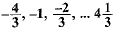Ans.
Here a = -4/3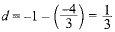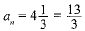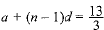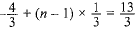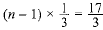⇒ n -1 = 17
⇒ n = 18
∵ n = 18 (even)
∴ Middle terms are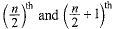i.e. 9th and 10th term
Now, sum = a9 + a10
= a + 8d + a + 9d
= 2 a + 17 d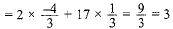So, the sum of two middle most terms = 3

Q.2. find the sum of the integers between 100 and 200 that are
(i) divisible by 9. (ii) not divisible by 9.
[Hint (ii): These numbers will be: Total numbers - Total numbers divisible by 9]
Ans.
(i) Numbers divisible by 9 between 100 and 200 are 108, 117, 126, ..., 198.
Here, a = 108, d = 9, an = 198
an = a + (n - 1) d
⇒ 198 = 108 + (n - 1)9
⇒ 198 = 108 + 9n - 9
⇒ 198 = 99 + 9n
⇒ 198 - 99 = 9n
⇒ 99/9 = n ⇒ 11 = n
and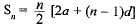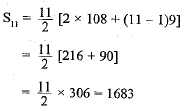(ii) Numbers between 100 and 200 are 101,102,..., 199
a = 101, d = 1, an = 199
∴ an = a + (n - 1 )d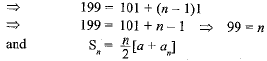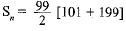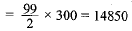∴  Sum of numbers not divisible by 9
= 14850 - 1683 = 13167.

Q.3. If the sum of first 6 terms of an AP is 36 and that of the first 16 terms is 256, find the sum of first 10 terms.
Ans.
∵ S6 = 36 and S16 = 256.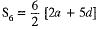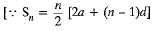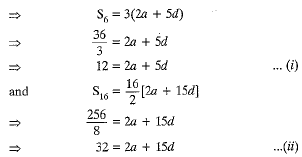Subtracting (i) and (ii), 2a + 5d = 12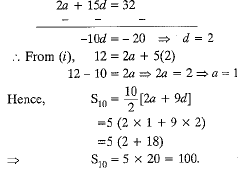Q.4. Kanika was given her pocket money on Jan 1st, 2008. She puts ₹ 1 on day 1, ₹ 2 on day 2, ₹ 3 on day 3, and continued doing so till the end of the month, from this money into her piggy bank. She also spent ₹ 204 of her pocket money, and found that at the end of the month she still had ₹ 100 with her. How much was her pocket money for the month?
Ans.
Here a = 1, d = 1 and a = 31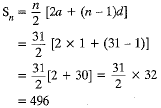∴ Piggy bank amount = ₹ 496
Amount spent = ₹ 204
Amount left = ₹ 100
Total pocket money = ₹ 800

Q.5. The sum of the first five terms of an AP and the sum of the first seven terms of the same AP is 167. If the sum of the first ten terms of this AP is 235, find the, sum of its first twenty terms.
Ans.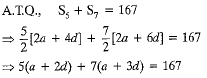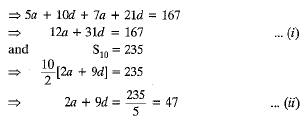Multiplying equation (ii) by 6 and then subtracting from
(i), we have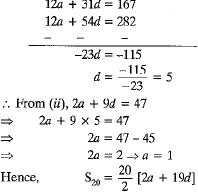= 10 [2 x 1 + 19 x 5]
= 10 [2 + 95] = 10 x 97 = 970

Q.6. The students of a school decided to beautify the school on the Annual Day by fixing colourful flags on the straight passage of the school. They have 27 flags to be fixed at intervals of every 2 m. The flags are stored at the position of the middle most flag. Ruchi was given the responsibility of placing the flags. Ruchi kept her books where the flags were stored. She could carry only one flag at a time. How much distance did she cover in completing this job and returning back to collect her books? What is the maximum distance she travelled carrying a flag?
Ans.
n - 27
Middle most term =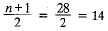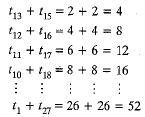Hence AP becomes 4, 8, 12, 16, ..... , 52
a = 4, d = 4, an = 52, n = 13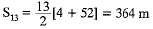and distance covered to collect the books = 364 m
∴ Total distance covered = 364 + 364 = 728 m
Maximum distance she travelled carrying a flag is 26 m.

Q.7. The ratio of the 11th term to the 18th term of an AP is 2 : 3 . Find the ratio of the 5th term to the 21st term, and also the ratio of the sum of the first five terms to the sum of the first 21 terms.
Ans.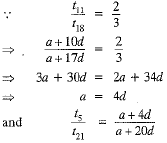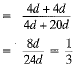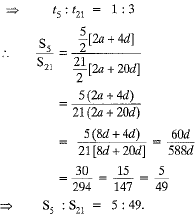Q.8. Show that the sum of an AP whose first term is a, the second term b and the last term c, is equal to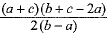Ans.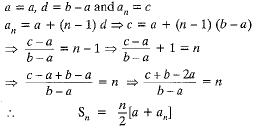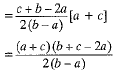## Mathematics (Maths) Class 10

172 videos|269 docs|103 tests

,

,

,

,

,

,

,

,

,

,

,

,

,

,

,

,

,

,

,

,

,

;